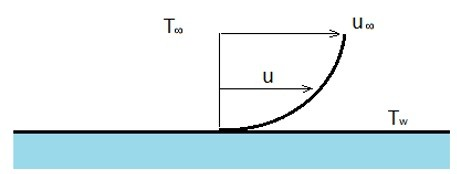# Convection

It is well known that if a hot object is placed in front of a fan it will cool faster than if it was left in stagnant air, or that it feels colder on a windy day than it does on a calm day. This is caused by convective heat transfer. Convective heat transfer is caused by fluid flowing over an object and either taking heat out of the object or possible transferring heat into the object. The rate of the heat transfer relates to how fast the fluid is flowing over the object, if the fluid is in a laminar or turbulent state, the thermal conductivity of the fluid, and the surface area of the object that the fluid is flowing over, all effect convection heat transfer.

In order to calculate the rate of heat transfer due to convection the following equation would be used. This equation is known as Newton’s law of cooling.

(Eq 1) $q = hA(T_w-T_∞)$

h = convective heat transfer coefficient

A = Surface Area exposed to the fluid flow

Tw = Temperature of object

T = Temperature of Fluid

I will show you in other sections how to calculate the convective heat transfer coefficient. This is dependent on the initial conditions of the fluid flow, what the fluid is, and if the fluid is turbulent or laminar.

Below is an example of convection caused by fluid flowing over a flat plate.From the above image it can be seen due to the viscous effects of the fluid the fluid flow will actually become zero directly at the wall. This means that the heat is only transferred by conduction. Due to this fact the convective heat transfer coefficient is sometimes called the film conductance because of its relationship to the thin stationary layer of fluid that is at the wall surface.

There are two different type of convective processes. They are forced convection, and natural or free convection. Natural convection happens when a heated plate is exposed to the ambient temperature of a room and there is no external source of movement of the fluid around the plate. Instead the air next to the plate moves due to density gradients caused by cold air coming in to replace the air that was warmed by the plate. Forced convection on the other hand occurs when the fluid is manipulated to cause it to move across the object. For example a fan blowing air across a flat plate, or water flowing through the plumbing of a heat exchanger.

## Example

Air is blowing over a plate that is has a surface area of .2 m2. The temperature of the surface of the plate is 60 degrees C. While the ambient temperature of the air is 20 degrees C. The convective heat transfer coefficient of the air moving over the plate is 60 W/m2-C. How much heat is the air pulling out of the plate?

(Eq 2) $q = (60)(0.2)(60-20) = 480W$